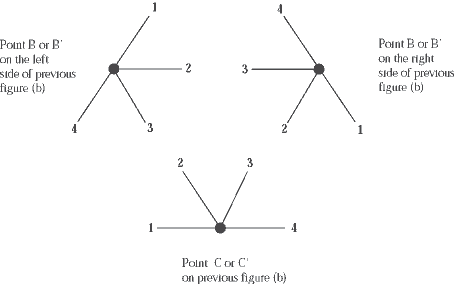HandsOn 30 - Random Walk on a Fractal

We now attempt to determine experimentally the average time it takes for a particle to move from one point to another on the Sierpinksi Gasket. The method is as follows: Roll a four-sided die, and move a random walker on the gasket. Keep count of how many steps the walker takes to move from one point to another point. By repeating this procedure, you will find the average time necessary to move specific distances.

Now for the details. "Time'' in this case means the same thing as the "total number of steps'' taken by the random walker. If it makes it easier for you, call the unit of time 1 second, and assume the walker takes 1 step per second. Then 10 steps take 10 seconds, 50 steps take 50 seconds, and so forth. The number of steps-the time-to go from one point to another will most likely be different for different trials because of the randomness of the process, based on the flip of a coin or the throw of a die. Hence we will try to predict the average times to go from one point to another. To emphasize that the times are averages, we use brackets. For example, the average time it takes to go from Point A to Point B is writtenTAB.

In the following experiment we use a 4-sided die (available in some game stores) to produce random numbers. To use such a die, roll it and look at the number which appears along the bottom edge of the die. This is the result of your roll.

 Q8.5: If you have only an ordinary 6-sided die, use four of the faces. That is, if a 5 or 6 comes up, ignore it and roll again. Speculate: Is this really the same as using a 4-sided die?Figure 8.3: Choosing the direction for the next step from labeled points in Figure 8.2(b). In each case, the direction is given by the number resulting from the roll of a 4-sided die.

To measure the average time it takes to go from one external vertex to another external point on the Sierpinski gasket (for example, from A to B' in Figure 8.2(a)), carry out the following steps. First, place your "walker'' (e.g., penny, pen top, thumb tack) at point A on the gasket. Flip a coin. If the coin comes up heads, move the walker down to the right. If the result is tails, move the walker down to the left. Now there are four possible directions for the next step. Roll the die. Move the walker according to the diagram in Figure 8.3. Select from the three possible orientations the one that fits your current position, and move the walker accordingly.

1. Find the average timeTAB'ñ(that is, the average number of random steps) required to move from point A to either of the two points labeled B' in Figure 8.2(a) or 8.2(b). Start with your walker at point A and tally the number of steps it takes to arrive at either point labeled B'. Record this number and repeat this trial 10 times. On each trial, record also the number of steps needed for the walker to arrive for the first time at point C'. Record only the number of steps prior to the first arrival at C'. Ignore any later returns to C'. Then continue with your walk until you arrive at either B'. (It is possible that the walker will not reach C' on a given trial. In that case, you won't have data for arrival at C' for that trial. That's all right.) Record your results on a data sheet.

2. The goal of this second task is to evaluate the average timeTAB''(= average number of random steps) to move from Point A to either of the points B'' in Figure 8.2(b). As in Task 1, for each trial start the walker at Point A and record the number of steps taken before arriving at either of the equivalent vertices labeled B''. Also record the number of steps necessary to first arrive at either point B' on each trial (this data can be used to supplement your results in Task 1). Carry out 10 such trials recording your results for each one on a data sheet.

3. Now analyze your data. Because 10 is a small number of trials, your average results may be very different from your neighbor's. You can obtain better results by averaging the results of your trials with those of the other members of the class.

 Q8.6: ComputeTAB', the average time it takes to move from point A to either point B'. Use the data from Task 1. Using the data from Task 2, computeTAB''. How do these two averages compare to what the movement would be like on a square grid?

 Q8.7: ComputeTC'B'. (Hint: You counted the number of steps necessary to arrive first at C' and then to arrive at either B'. For each trial where you passed through C', subtract the former from the latter to find the number of steps needed to move from C' to either B'.

Q8.8: From Figure 8.2(a), see if you can justify the following equation:TAB'= 1 +TBB'.
(8.1)
Here we have written 1 forTAB, the time needed to go from A to either point B in Figure 8.2(a). Use this equation to compute áTBB'ñ from your data. (With two more such equations, it is possible to solve exactly for the average first arrival time at an external vertex on a Sierpinski gasket.

Previous: 8.1 - Random Walk on a Fractal

Next: 8.2 - The Power Law for a Random Walker on a Sierpinski Gasket